Introduction

This package contains functions that post-process an output from semPlot::semPaths(), to help users to customize the appearance of the graphs generated by semPlot::semPaths().

The following sections were written to be self-contained, with some elements repeated, such that each of them can be read individually.

Mark all parameter estimates by asterisks based on p-Value: mark_sig

Let us consider a simple path analysis model:

library(lavaan)
mod_pa <-
'x1 ~~ x2
x3 ~  x1 + x2
x4 ~  x1 + x3
'
fit_pa <- lavaan::sem(mod_pa, pa_example)
parameterEstimates(fit_pa)
#>   lhs op rhs   est    se     z pvalue ci.lower ci.upper
#> 1  x1 ~~  x2 0.005 0.097 0.054  0.957   -0.186    0.196
#> 2  x3  ~  x1 0.537 0.097 5.551  0.000    0.348    0.727
#> 3  x3  ~  x2 0.376 0.093 4.050  0.000    0.194    0.557
#> 4  x4  ~  x1 0.111 0.127 0.875  0.382   -0.138    0.361
#> 5  x4  ~  x3 0.629 0.108 5.801  0.000    0.416    0.841
#> 6  x3 ~~  x3 0.874 0.124 7.071  0.000    0.632    1.117
#> 7  x4 ~~  x4 1.194 0.169 7.071  0.000    0.863    1.525
#> 8  x1 ~~  x1 0.933 0.132 7.071  0.000    0.674    1.192
#> 9  x2 ~~  x2 1.017 0.144 7.071  0.000    0.735    1.298

This is the plot from semPaths.

library(semPlot)
m <- matrix(c("x1",   NA,  NA,   NA,
NA, "x3",  NA, "x4",
"x2",   NA,  NA,   NA), byrow = TRUE, 3, 4)
p_pa <- semPaths(fit_pa, whatLabels = "est",
sizeMan = 10,
edge.label.cex = 1.15,
style = "ram",
nCharNodes = 0, nCharEdges = 0,
layout = m)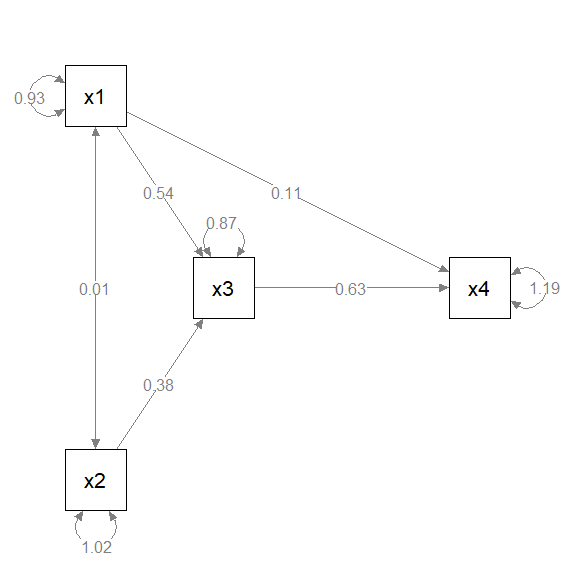We know from the lavaan::lavaan() output that some paths are significant and some are not. In some disciplines, asterisks are conventionally added indicate this. However, semPlot::semPaths() does not do this. We can use mark_sig() to add asterisks based on the p-values of the free parameters.

library(semptools)
p_pa2 <- mark_sig(p_pa, fit_pa)
plot(p_pa2)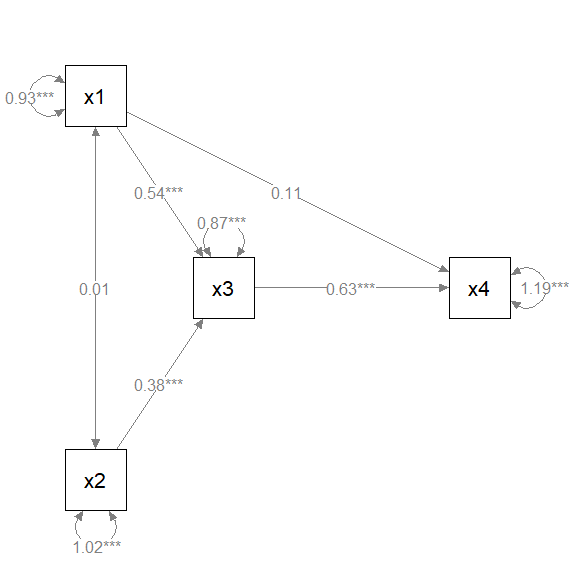The first argument, semPaths_plot, is the output from semPaths::semPlot(). The second argument, object, is the lavaan::lavaan() output used to generate the plot. This output is needed to extract the p-values.

The default labels follow the common convention: “*” for p less than .05, “**” for p less than .01, and “***” for p less than .001. This can be changed by the argument alpha (this must be named as the it is not the second argument). E.g.:

p_pa3 <- mark_sig(p_pa, fit_pa, alpha = c("(n.s.)" = 1.00, "*" = .01))
plot(p_pa3)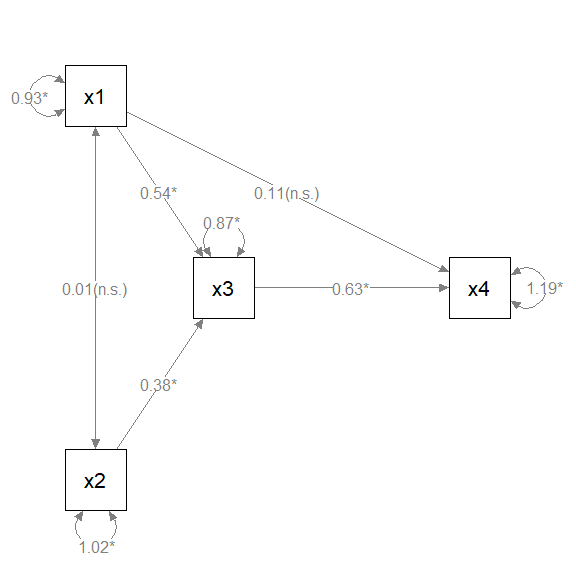Add standard error estimates to parameter estimates: mark_se

Let us consider a simple path analysis model:

library(lavaan)
mod_pa <-
'x1 ~~ x2
x3 ~  x1 + x2
x4 ~  x1 + x3
'
fit_pa <- lavaan::sem(mod_pa, pa_example)
parameterEstimates(fit_pa)
#>   lhs op rhs   est    se     z pvalue ci.lower ci.upper
#> 1  x1 ~~  x2 0.005 0.097 0.054  0.957   -0.186    0.196
#> 2  x3  ~  x1 0.537 0.097 5.551  0.000    0.348    0.727
#> 3  x3  ~  x2 0.376 0.093 4.050  0.000    0.194    0.557
#> 4  x4  ~  x1 0.111 0.127 0.875  0.382   -0.138    0.361
#> 5  x4  ~  x3 0.629 0.108 5.801  0.000    0.416    0.841
#> 6  x3 ~~  x3 0.874 0.124 7.071  0.000    0.632    1.117
#> 7  x4 ~~  x4 1.194 0.169 7.071  0.000    0.863    1.525
#> 8  x1 ~~  x1 0.933 0.132 7.071  0.000    0.674    1.192
#> 9  x2 ~~  x2 1.017 0.144 7.071  0.000    0.735    1.298

This is the plot from semPlot::semPaths().

library(semPlot)
m <- matrix(c("x1",   NA,  NA,   NA,
NA, "x3",  NA, "x4",
"x2",   NA,  NA,   NA), byrow = TRUE, 3, 4)
p_pa <- semPaths(fit_pa, whatLabels = "est",
sizeMan = 10,
edge.label.cex = 1.15,
style = "ram",
nCharNodes = 0, nCharEdges = 0,
layout = m)We can use mark_se() to add the standard errors for the parameter estimates:

library(semptools)
p_pa2 <- mark_se(p_pa, fit_pa)
plot(p_pa2)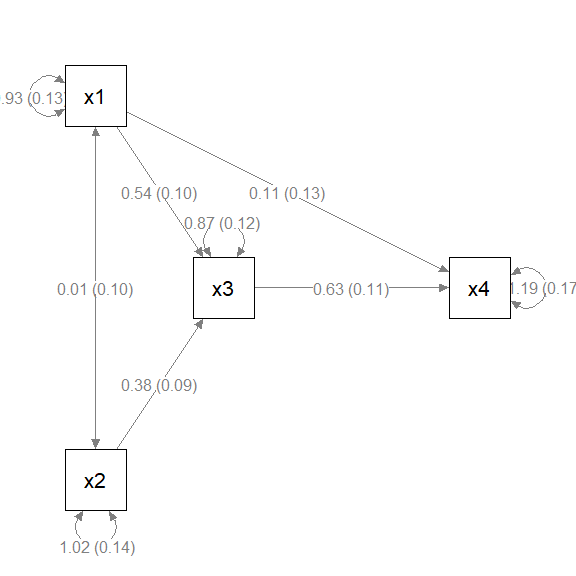The first argument, semPaths_plot, is the output from semPaths::semPlot(). The second argument, object, is the lavaan::lavaan() output used to generate the plot. This output is needed to extra the standard errors.

By default, the standard errors are enclosed by parentheses and appended to the parameter estimates, separated by one space. The argument sep can be used to use another separator. For example, if "\n" is used, the standard errors will be displayed below the corresponding parameter estimates.

p_pa2 <- mark_se(p_pa, fit_pa, sep = "\n")
plot(p_pa2)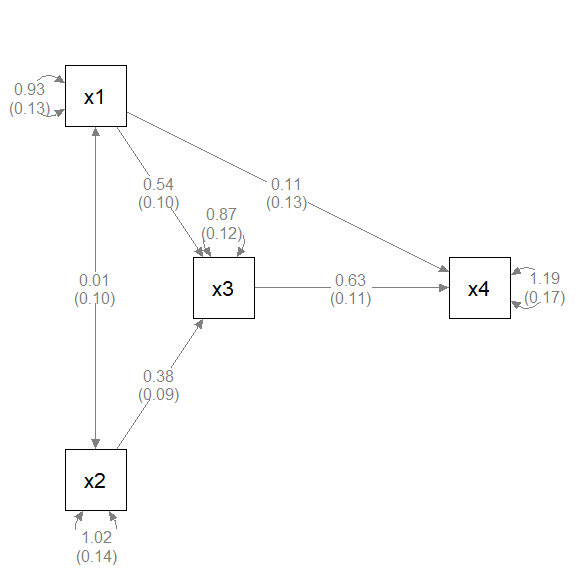Rotate the residuals of selected variables: rotate_resid

Let us consider a simple path analysis model:

library(lavaan)
mod_pa <-
'x1 ~~ x2
x3 ~  x1 + x2
x4 ~  x1 + x3
'
fit_pa <- lavaan::sem(mod_pa, pa_example)

This is the plot from semPlot::semPaths().

library(semPlot)
m <- matrix(c("x1",   NA,  NA,   NA,
NA, "x3",  NA, "x4",
"x2",   NA,  NA,   NA), byrow = TRUE, 3, 4)
p_pa <- semPaths(fit_pa, whatLabels = "est",
sizeMan = 10,
edge.label.cex = 1.15,
style = "ram",
nCharNodes = 0, nCharEdges = 0,
layout = m)Suppose we want to rotate the residuals of some variables to improve readability.

• For x3, we want to place the residual to top-right corner.

• For x4, we want to place the residual to the top-left corner.

• For x2, we want to place the residual to the left.

We first need to decide the angle of placement, in degrees.

Top is 0 degree. Clockwise position is positive, and anticlockwise position is negative.

Therefore, top-right is 45, top-left is -45, and left is -90.

We then use rotate_resid() to post-process the semPlot::semPaths() output. The first argument, semPaths_plot, is the semPlot::semPaths() output. The second argument, rotate_resid_list, is the vector to specify how the residuals should be rotated. The name is the node for which the residual will be rotated, and the value is the degree of rotation. For example, to achieve the results described above, the vector is c(x3 = 45, x4 = -45, x2 = -90):

library(semptools)
my_rotate_resid_list <- c(x3 =  45,
x4 = -45,
x2 = -90)
p_pa3 <- rotate_resid(p_pa, my_rotate_resid_list)
plot(p_pa3)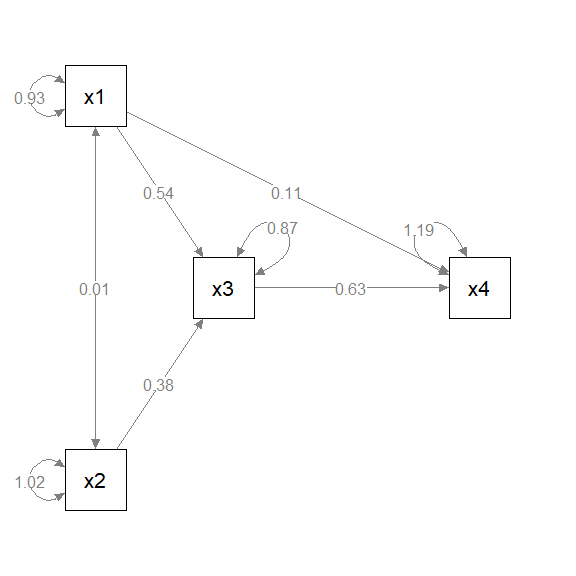(Note: This function accepts named vectors since version 0.2.8. Lists of named list are still supported but not suggested. Please see ?rotate_resid on how to use lists of named list.)

Set the curve attributes of selected arrows: set_curve

Let us consider a simple path analysis model:

library(lavaan)
mod_pa <-
'x1 ~~ x2
x3 ~  x1 + x2
x4 ~  x1 + x3
'
fit_pa <- lavaan::sem(mod_pa, pa_example)

This is the plot from semPaths.

library(semPlot)
m <- matrix(c("x1",   NA,  NA,   NA,
NA, "x3",  NA, "x4",
"x2",   NA,  NA,   NA), byrow = TRUE, 3, 4)
p_pa <- semPaths(fit_pa, whatLabels = "est",
sizeMan = 10,
edge.label.cex = 1.15,
style = "ram",
nCharNodes = 0, nCharEdges = 0,
layout = m)Suppose we want to change the curvature of these two arrows (edges):

• Have the x1 ~~ x2 covariance curved “away” from the center.

• Have the x4 ~ x1 path curved upward.

We then use set_curve() to post-process the semPlot::semPaths() output. The first argument, semPaths_plot, is the semPlot::semPaths() output. The second argument, curve_list, is the list to specify the new curvature of the selected arrows.

The “name” of each element is of the same form as lhs-op-rhs as in lavaan::lavaan() model syntax. In lavaan, y ~ x denotes an arrow from x to y. Therefore, if we want to change the curvature of the path from x to y to -3, then the element is "y ~ x" = -3. Note that whether ~ or ~~ is used does not matter.

To achieve the changes described above, we can use c("x2 ~~ x1" = -3, "x4 ~ x1" = 2), as shown below:

my_curve_list <- c("x2 ~~ x1" = -3,
"x4  ~ x1" =  2)
p_pa3 <- set_curve(p_pa, my_curve_list)
plot(p_pa3)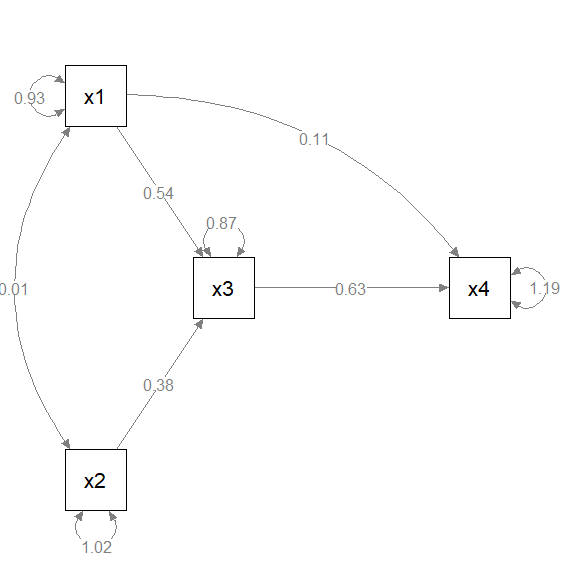Note that the meaning of the value depends on which variable is in the from field and which variable is in the to field. Therefore, "x2 ~~ x1" = -3 and "x1 ~~ x2" = -3 are two different changes. If we treat the from variable as the back and the to variable as the front, then a positive number bends the line to left, and a negative number bends the line to the right.

It is not easy to decide what the value should be used to set the curve. Trial and error is needed for complicated models. The curve attributes of the corresponding arrows of the qgraph object will be updated.

(Note: This function accepts named vectors since version 0.2.8. Lists of named list are still supported but not suggested. Please see ?set_curve on how to use lists of named list.)

Set the positions of parameters of selected arrows: set_edge_label_position

Let us consider a simple path analysis model:

library(lavaan)
mod_pa <-
'x1 ~~ x2
x3 ~  x1 + x2
x4 ~  x1 + x3
'
fit_pa <- lavaan::sem(mod_pa, pa_example)

This is the plot from semPlot::semPaths().

library(semPlot)
m <- matrix(c("x1",   NA,  NA,   NA,
NA, "x3",  NA, "x4",
"x2",   NA,  NA,   NA), byrow = TRUE, 3, 4)
p_pa <- semPaths(fit_pa, whatLabels = "est",
sizeMan = 10,
edge.label.cex = 1.15,
style = "ram",
nCharNodes = 0, nCharEdges = 0,
layout = m)Suppose we want to move the parameter estimates this way:

• For the x4 ~ x1 path, move the parameter estimates closer to x4.

• For the x3 ~ x1 path, move the parameter estimates closer to x1.

• For the x3 ~ x2 path, move the parameter estimates closer to x2.

We can use set_edge_label_position() to post-process the semPlot::semPaths output. The first argument, semPaths_plot, is the semPlot::semPaths() output. The second argument, position_list, is the list to specify the new position of the selected arrows.

We can use a named vector to specify the changes. The “name” of each element is of the same form as lhs-op-rhs as in lavaan::lavaan() model syntax. In lavaan, y ~ x denotes an arrow from x to y. Therefore, if we want to change the curvature of the path from x to y to -3, then the element is "y ~ x" = -3. Note that whether ~ or ~~ is used does not matter.

Therefore, the changes described above can be specified by c("x2 ~~ x1" = -3, "x4 ~ x1" = 2), as shown below:

library(semptools)
my_position_list <- c("x3 ~ x1" = .25,
"x3 ~ x2" = .25,
"x4 ~ x1" = .75)
p_pa3 <- set_edge_label_position(p_pa, my_position_list)
plot(p_pa3)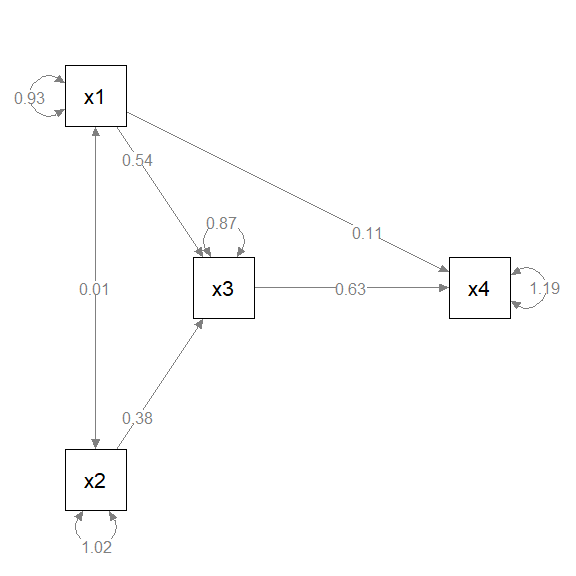(Note: This function accept named vectors since version 0.2.8. Lists of named list are still supported but not suggested. Please see ?set_edge_label_position on how to use lists of named list.)

Change one or more node labels: change_node_label

semPlot::semPaths() supports changing the labels of nodes when generating a plot through the argument nodeLabels. However, if we want to use functions such as mark_sig() or mark_se(), which require information from the original results from the original lavaan output, then we cannot use nodeLabels because these functions do not (yet) know how to map a user-defined label to the variables in the lavaan output.

One solution is to use semptools functions to process the qgraph generated by semPlot::semPaths(), and change the node labels in last step to create the final plot. This can be done by change_node_label().

Let us consider a simple path analysis model in which we use marg_sig() to add asterisks to denote significant parameters:

library(lavaan)
library(semPlot)
library(semptools)
mod_pa <-
'x1 ~~ x2
x3 ~  x1 + x2
x4 ~  x1 + x3
'
fit_pa <- lavaan::sem(mod_pa, pa_example)
m <- matrix(c("x1",   NA,  NA,   NA,
NA, "x3",  NA, "x4",
"x2",   NA,  NA,   NA), byrow = TRUE, 3, 4)
p_pa <- semPaths(fit_pa, whatLabels = "est",
sizeMan = 10,
edge.label.cex = 1.15,
style = "ram",
nCharNodes = 0, nCharEdges = 0,
layout = m)p_pa2 <- mark_sig(p_pa, fit_pa, alpha = c("(n.s.)" = 1.00, "*" = .01))
plot(p_pa2)Suppose we want change x1, x2, x3, and x4 to Attitude, SbjNorm, Intention, and Behavior, we process the graph, p_pa2 above, by change_node_label() as below:

p_pa3 <- change_node_label(p_pa2,
c(x1 = "Attitude",
x2 = "SbjNorm",
x3 = "Intention",
x4 = "Behavior"),
label.cex = 1.1)
plot(p_pa3)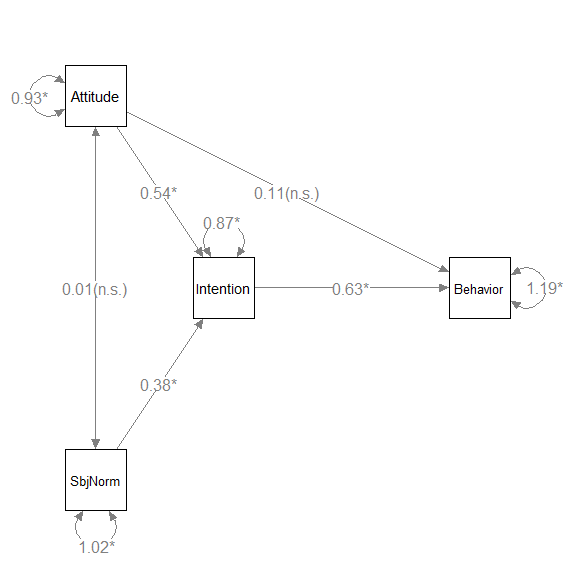The second argument can be a named vector or a named list. The name of each element is the original label (e.g., x1 in this example), and the value is the new label (e.g., "Attitude" for x1). Only the labels of named nodes will be changed.

Note that usually we also set the label.cex argument, which is identical to the same argument in semPlot::semPaths() because the new labels might not fit the nodes.

Using pipe-operator

All the functions support the %>% operator from magrittr or the native pipe operator |> available since R 4.1.x. Therefore, we can chain the post-processing.

library(lavaan)
mod_pa <-
'x1 ~~ x2
x3 ~  x1 + x2
x4 ~  x1 + x3
'
fit_pa <- lavaan::sem(mod_pa, pa_example)

This is the initial plot:

library(semPlot)
m <- matrix(c("x1",   NA,  NA,   NA,
NA, "x3",  NA, "x4",
"x2",   NA,  NA,   NA), byrow = TRUE, 3, 4)
p_pa <- semPaths(fit_pa, whatLabels = "est",
sizeMan = 10,
edge.label.cex = 1.15,
style = "ram",
nCharNodes = 0, nCharEdges = 0,
layout = m)We will do this:

• Change the curvature of x1 ~~ x2

• Rotate the residuals of x1, x2, x3, and x4,

• Add asterisks to denote significant test results

• Move the parameter estimate of the x4 ~ x1 path closer to x4.

my_position_list <- c("x4 ~ x1" = .75)
my_curve_list <- c("x2 ~ x1" = -2)
my_rotate_resid_list <- c(x1 = 0, x2 = 180, x3 = 140, x4 = 140)
my_position_list <- c("x4 ~ x1" = .65)
# If R version 4.1.0 or above
p_pa3 <- p_pa |> set_curve(my_curve_list) |>
rotate_resid(my_rotate_resid_list) |>
mark_sig(fit_pa) |>
mark_se(fit_pa, sep = "\n") |>
set_edge_label_position(my_position_list)
plot(p_pa3)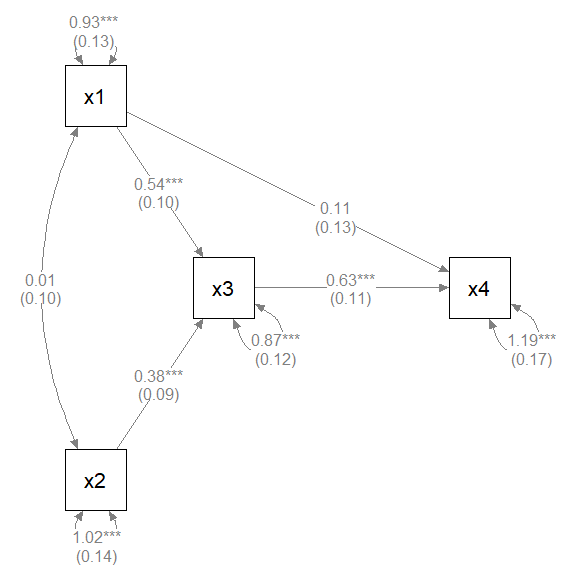For most of the functions, the necessary argument beside the semPlot::semPaths output, if any, is the second element. Therefore, they can be included as unnamed arguments. For the third and other optional arguments, such as sep for mark_se(), it is better to name them.

Limitations

• Currently, if a function needs the SEM output, only lavaan output is supported.